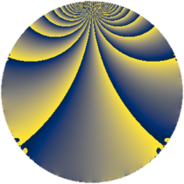# Properties

 Label 231.2.nLevel $231$ Weight $2$ Character orbit 231.n Rep. character $\chi_{231}(89,\cdot)$ Character field $\Q(\zeta_{6})$ Dimension $52$ Newform subspaces $1$ Sturm bound $64$ Trace bound $0$

# Learn more about

## Defining parameters

 Level: $$N$$ $$=$$ $$231 = 3 \cdot 7 \cdot 11$$ Weight: $$k$$ $$=$$ $$2$$ Character orbit: $$[\chi]$$ $$=$$ 231.n (of order $$6$$ and degree $$2$$) Character conductor: $$\operatorname{cond}(\chi)$$ $$=$$ $$21$$ Character field: $$\Q(\zeta_{6})$$ Newform subspaces: $$1$$ Sturm bound: $$64$$ Trace bound: $$0$$

## Dimensions

The following table gives the dimensions of various subspaces of $$M_{2}(231, [\chi])$$.

Total New Old
Modular forms 72 52 20
Cusp forms 56 52 4
Eisenstein series 16 0 16

## Trace form

 $$52q + 24q^{4} - 6q^{7} - 4q^{9} + O(q^{10})$$ $$52q + 24q^{4} - 6q^{7} - 4q^{9} - 30q^{12} - 4q^{15} - 20q^{16} - 18q^{18} + 6q^{19} - 2q^{21} + 36q^{24} - 22q^{25} - 40q^{28} - 20q^{30} - 54q^{31} + 36q^{36} + 2q^{37} + 20q^{39} + 60q^{40} - 36q^{42} + 36q^{43} - 54q^{45} + 4q^{46} + 22q^{49} - 16q^{51} + 12q^{52} + 66q^{54} - 44q^{57} - 16q^{58} + 20q^{60} - 36q^{61} - 24q^{63} - 48q^{64} - 30q^{66} - 6q^{67} - 116q^{70} + 36q^{72} - 6q^{73} + 72q^{75} + 112q^{78} + 14q^{79} + 52q^{81} - 84q^{82} + 8q^{84} + 8q^{85} + 18q^{87} - 12q^{88} + 46q^{91} + 6q^{93} + 48q^{94} - 18q^{96} + O(q^{100})$$

## Decomposition of $$S_{2}^{\mathrm{new}}(231, [\chi])$$ into newform subspaces

Label Dim. $$A$$ Field CM Traces $q$-expansion
$$a_2$$ $$a_3$$ $$a_5$$ $$a_7$$
231.2.n.a $$52$$ $$1.845$$ None $$0$$ $$0$$ $$0$$ $$-6$$

## Decomposition of $$S_{2}^{\mathrm{old}}(231, [\chi])$$ into lower level spaces

$$S_{2}^{\mathrm{old}}(231, [\chi]) \cong$$ $$S_{2}^{\mathrm{new}}(21, [\chi])$$$$^{\oplus 2}$$